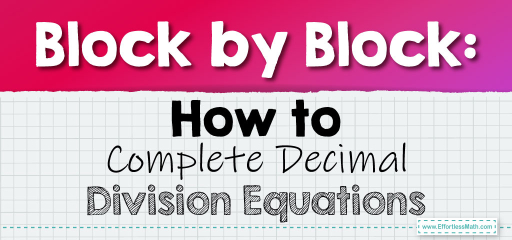# Block by Block: How to Complete Decimal Division Equations

Using visual aids like blocks can make understanding mathematical concepts, especially decimals, much more intuitive. When dividing decimals, envisioning blocks can help us grasp the concept better. Let's explore how to approach this using blocks.Visualizing with Blocks:

Imagine each block represents a unit. When we talk about decimals, we can think of them as parts of a block. For instance, if a block represents $$1$$, then $$0.1$$ would be a tenth of that block.

## Dividing Decimals Using Blocks: Finish the Equation

### Example 1:

Complete the division sentence: $$0.4 \div \_ = 0.2$$.

Solution Process:

1. Visualize $$0.4$$ as four-tenths of a block.

2. Determine how many times you need to divide this portion to get $$0.2$$ or two-tenths of a block.

Using blocks, you can determine that $$0.4$$ divided by $$2$$ gives $$0.2$$.

The Absolute Best Book for 5th Grade Students

### Example 2:

Complete the division sentence: $$0.6 \div \_ = 0.3$$.

Solution Process:

1. Visualize $$0.6$$ as six-tenths of a block.

2. Determine how many times you need to divide this portion to get $$0.3$$ or three-tenths of a block.

Using blocks, you can determine that $$0.6$$ divided by $$2$$ gives $$0.3$$.

Using blocks to visualize decimal division provides a tangible way to understand the concept. It bridges the gap between abstract numbers and real-world representations, making the learning process engaging and effective. Whether you’re a student, teacher, or someone looking to understand decimals better, using blocks as a visual aid can be a game-changer. So, the next time you’re faced with a decimal division problem, think in blocks and watch the numbers come to life!

### Practice Questions:

1. Complete the division sentence: $$0.5 \div \_ = 0.25$$.

2. Determine the missing divisor: $$0.9 \div \_ = 0.3$$.

3. Complete the division sentence: $$0.8 \div \_ = 0.4$$.

4. Determine the missing divisor: $$0.7 \div \_ = 0.1$$.

5. Complete the division sentence: $$0.3 \div \_ = 0.1$$.

A Perfect Book for Grade 5 Math Word Problems!

1. $$2$$

2. $$3$$

3. $$2$$

4. $$7$$

5. $$3$$

The Best Math Books for Elementary Students

### What people say about "Block by Block: How to Complete Decimal Division Equations - Effortless Math: We Help Students Learn to LOVE Mathematics"?

No one replied yet.

X
51% OFF

Limited time only!

Save Over 51%

SAVE $15 It was$29.99 now it is \$14.99